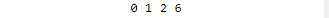Related Articles
Non-Repeating Elements of a given array using Multithreaded program
• Last Updated : 09 Dec, 2020

Given an array arr[] of size N and an integer T representing the count of threads, the task is to find all non-repeating array elements using multithreading.

Examples:

Input: arr[] = { 1, 0, 5, 5, 2}, T = 3
Output: 0 1 2
Explanation:
The frequency of 0 in the array arr[] is 1.
The frequency of 1 in the array arr[] is 1.
The frequency of 2 in the array arr[] is 1.
Therefore, the required output is 0 1 2

Input: arr[] = { 1, 1, 5, 5, 2, 4 }, T = 3
Output: 2 4
Explanation:
The frequency of 2 in the array arr[] is 1.
The frequency of 4 in the array arr[] is 1.
Therefore, the required output is 2 4

## Recommended: Please try your approach on {IDE} first, before moving on to the solution.

Approach: The idea is to use the pthread library available in C++ to create multiple threads for concurrent process flow and perform multiple operations( pthread create, pthread join , lock, etc) in multithreaded program. Follow the steps below to solve the problem:

• Divide the array into T subarrays, such that each subarray of size N / T will be executed in a single thread.
• Initialize a Map, say mp, to store the frequencies of each array element.
• Create a pthread_mutex_lock, say lock1, to ensure that all threads do not trip over each other and corrupt the Map container.
• Define a function func() for executing the body of a thread. This function is often called the kernel of the thread and is provided during thread creation.
• Traverse through the given range as an argument to the function func() in the array arr[] and increment the frequency of the array element which is encountered.
• Iterate over the range [0, T] and create a thread by calling pthread_create() function and store it in the thread[i]
• While each thread performs their individual tasks, the main() function will need to wait till each of the threads finish their work.
• Use pthread_join() function for waiting till each thread finishes executing function func()
• Iterate over the range [0, T] and call pthread_create() function for each thread[i]
• Finally, traverse the map mp and print the element occurring only once.

Below is the implementation of the above approach:

## C++

 `// C++ program to implement``// the above approach`` ` `#include ``#include ``using` `namespace` `std;`` ` `// Structure of subarray ``// of the array``struct` `range_info {`` ` `    ``// Stores start index``    ``// of the subarray``    ``int` `start;`` ` `    ``// Stores end index``    ``// of the subarray``    ``int` `end;`` ` `    ``// Stores pointer to the``    ``// first array element``    ``int``* a;``};`` ` ` ` `// Declaring map, and mutex for``// thread exclusion(lock)``map<``int``, ``int``> mp;``pthread_mutex_t lock1;`` ` ` ` `// Function performed by every thread to``// count the frequency of array elements``// in current subarray``void``* func(``void``* arg)``{``    ``// Taking a lock so that threads``    ``// do not overlap each other``    ``pthread_mutex_lock(&lock1);``     ` `     ` `    ``// Initalize range_info ``    ``// for each thread``    ``struct` `range_info* rptr ``    ``= (``struct` `range_info*)arg;`` ` ` ` `    ``// Thread is going through the array``    ``// to check and update the map``    ``for` `(``int` `i = rptr->start; ``            ``i <= rptr->end; i++) {``                     ` `         ` `        ``// Stores iterator to the map         ``        ``map<``int``, ``int``>::iterator it;``         ` `         ` `        ``// Update it``        ``it = mp.find(rptr->a[i]);``         ` `         ` `        ``// If rptr->a[i] not found``        ``// in map mp``        ``if` `(it == mp.end()) {``             ` `             ` `            ``// Insert rptr->a[i] with ``            ``// frequency 1``            ``mp.insert({ rptr->a[i], 1 });``        ``}``        ``else` `{``             ` `             ` `            ``// Update frequency of``            ``// current element``            ``it->second++;``        ``}``    ``}``     ` ` ` `    ``// Thread releases the lock``    ``pthread_mutex_unlock(&lock1);``    ``return` `NULL;``}`` ` ` ` `// Function to find the unique``// numbers in the array``void` `numbers_occuring_once(``int` `arr[],``                        ``int` `N, ``int` `T)``{``    ``// Stores all threads``    ``pthread_t threads[T];`` ` `    ``// Stores start index of``    ``// first thread``    ``int` `spos = 0;`` ` `    ``// Stores last index ``    ``// of the first thread``    ``int` `epos = spos + (N / T) - 1;`` ` `    ``// Traverse each thread``    ``for` `(``int` `i = 0; i < T; i++) {`` ` `        ``// Initalize range_info for``        ``// current thread``        ``struct` `range_info* rptr``            ``= (``struct` `range_info*)``malloc``(``                ``sizeof``(``struct` `range_info));`` ` `        ``// Update start index of``        ``// current subarray     ``        ``rptr->start = spos;`` ` `        ``// Stores end index of``        ``// current subarray``        ``rptr->end = epos;`` ` `        ``// Update pointer to the first``        ``// element of the array``        ``rptr->a = arr;``         ` `         ` `        ``// creating each thread with ``        ``// appropriate parameters``        ``int` `a``        ``= pthread_create(&threads[i], NULL,``                        ``func, (``void``*)(rptr));``                         ` `                         ` `        ``// updating the parameters ``        ``// for the next thread``        ``spos = epos + 1;``        ``epos = spos + (N / T) - 1;``        ``if` `(i == T - 2) {``            ``epos = N - 1;``        ``}``    ``}`` ` `    ``// Waiting for threads to finish``    ``for` `(``int` `i = 0; i < T; i++) {``        ``int` `rc ``        ``= pthread_join(threads[i], NULL);``    ``}`` ` `    ``// Traverse the map``    ``for` `(``auto` `it: mp) {``                 ` `                 ` `                                     ` `    ``// If frequency of current ``    ``// element is 1                             ``        ``if` `(it.second == 1) {`` ` `            ``// Print the element``            ``cout << it.first << ``" "``;``        ``}``    ``}``}`` ` ` ` `// Drivers Code``int` `main()``{``    ``// initializing the mutex lock ``    ``pthread_mutex_init(&lock1, NULL);``    ``int` `T = 3;``    ``int` `arr[] = { 1, 0, 5, 5, 2, 6 };``    ``int` `N = ``sizeof``(arr) / ``sizeof``(arr);``    ``numbers_occuring_once(arr, N, T);``}`
Output:
``````

Time Complexity: O(N * log(N))
Auxiliary Space: O(N)

Note: It is recommended to execute the program in a Linux based system using the following command: